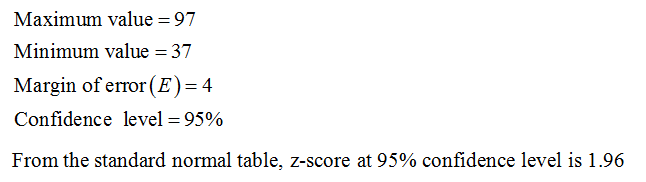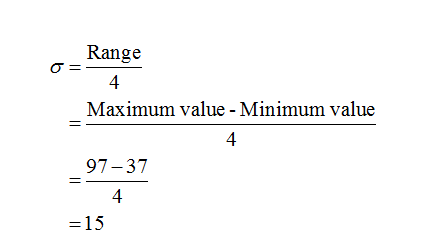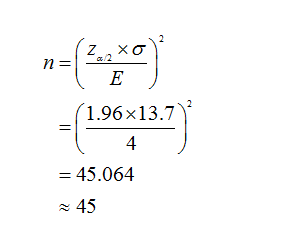# The pulse rates of 161 randomly selected adult males vary from a low of37 bpm to a high of 97 bpm. Find the minimum sample size required to estimate the mean pulse rate of adult males. Assume that we want 95​%confidence that the sample mean is within 4 bpm of the population mean. Complete parts​ (a) through​ (c) below.a. Find the sample size using the range rule of thumb to estimate σ.Assume that σ=13.7 bpm, based on the value s=13.7 bpm from the sample of 161male pulse rates.c. Compare the results from parts​ (a) and​ (b). Which result is likely to be​ better?

Question
1 views
The pulse rates of 161 randomly selected adult males vary from a low of
37 bpm to a high of 97 bpm. Find the minimum sample size required to estimate the mean pulse rate of adult males. Assume that we want 95​%
confidence that the sample mean is within 4 bpm of the population mean. Complete parts​ (a) through​ (c) below.
a. Find the sample size using the range rule of thumb to estimate σ.Assume that σ=13.7 bpm, based on the value s=13.7 bpm from the sample of 161
male pulse rates.
c. Compare the results from parts​ (a) and​ (b). Which result is likely to be​ better?
check_circle

Step 1

The provided information are:Step 2

(a)

To compute the sample size, standard deviation is required which can be calculated as:Now, the sample size can be calculated as:Step 3

(b)

Given:

σ=13.7

The sample size can be calculated as:...

### Want to see the full answer?

See Solution

#### Want to see this answer and more?

Solutions are written by subject experts who are available 24/7. Questions are typically answered within 1 hour.*

See Solution
*Response times may vary by subject and question.
Tagged in